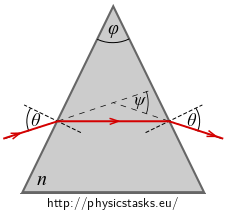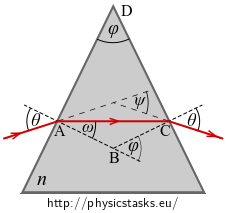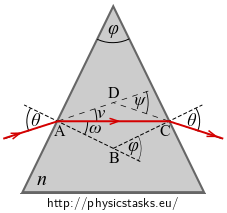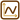## Index of Refraction of a Prism

### Task number: 2326We made a measurement to determine the refractive index $$n$$ of glass of a triangular prism as shown in the figure on the right.

We let a narrow beam of light hit the first boundary at an angle $$\theta$$ such that it was refracted on the second boundary at the same angle $$\theta$$.

We found out that the angle of deviation - which is the total deflection of a ray when passing through a prism – is $$\psi$$.

Find a relation which we can use to determine the unknown index of refraction $$n$$ from the measured values $$\varphi,\psi$$.

• #### Theory

When the ray is refracted on a boundary of two optical media (a and b) we can use Snell’s law of refraction:

$\frac{\sin \alpha}{\sin \beta} = \frac{n_b}{n_a},$

where $$\alpha$$ is the angle of incidence, $$\beta$$ the angle of refraction and $$n_a, n_b$$ the indices of refraction of the optical media.

• #### Hint 1 – law of refraction

We aim to determine the dependence of the index of refraction $$n$$ on angles $$\varphi$$ and $$\psi$$. We can see the relation between the index of refraction and the angles from the law of refraction on the boundary – write it.

• #### Hint 2 – expressing ω

We expressed the dependence of the unknown index of refraction $$n$$ on angles $$\theta, \omega$$ in equation (1). Although our assignment is to find the dependence on the measured values $$\varphi, \psi$$.

Express angle $$\omega$$ using measured angles $$\varphi, \psi$$.

• #### Hint 3 – expressing θ

We expressed the dependence of the unknown index of refraction $$n$$ on angles $$\theta, \omega$$ in equation (1). However, our assignment is to find the dependence on the measured values $$\varphi, \psi$$.

Express angle $$\theta$$ using the measured angles $$\varphi, \psi$$.

• #### Hint 4 – final expression

Substitute the relations (2) and (5) into the law of refraction (1).

• #### Complete solution

When the ray is refracted on a boundary of two optical media (a and b) we can use Snell’s law of refraction:

$\frac{\sin \alpha}{\sin \beta} = \frac{n_b}{n_a},$

where $$\alpha$$ is the angle of incidence, $$\beta$$ the angle of refraction and $$n_a, n_b$$ the indices of refraction of the optical media.

In our case it holds that:

$\frac{\sin\theta}{\sin\omega} = \frac{n}{1}$

We know from the task assignment that we want to determine the dependence of the index of refraction $$n$$ on angles $$\varphi$$ and $$\psi$$.

We will express angle $$\omega$$ using the measured angles.Size of angle $$\varphi$$ is the same as size of the external angle at the vertex B.

Sum of the internal angles of any rectangle, thus also rectangle ABCD, is 360°. Angles at vertices A and C are right angles. Angle at the vertex B is $$180° - \varphi$$. It follows that the adjacent angle at vertex B is equal to $$\varphi$$.

It holds for the isosceles $$\Delta ABC$$ that:

$\varphi = \omega + \omega,$

thus:

$\omega = \frac{\varphi}{2}.$

We will express angle $$\theta$$ using the measured angles.It holds for angles $$\theta, \nu, \omega$$ with vertex at point $$\mathrm{A}$$ that:

$\theta = \nu + \omega.$

Size of the external angle $$\psi$$ at vertex $$D$$ in $$\Delta ACD$$ is equal to sum of the internal angles at the other vertices. Because it is an isosceles triangle it holds that:

$\psi = \nu + \nu.$

Thus:

$\nu = \frac{\psi}{2}.$

Substituting we get:

$\theta = \nu + \omega = \frac{\psi+\varphi}{2}.$

Substituting the expressions of $$\varphi$$ and $$\psi$$ into the formula for calculating the index of refraction $$n$$ we get the relation which we wanted to find:

$n=\frac{\sin \frac{\psi + \varphi}{2} }{\sin\frac{\varphi}{2}}.$
$n=\frac{\sin \frac{\psi + \varphi}{2} }{\sin\frac{\varphi}{2}}.$